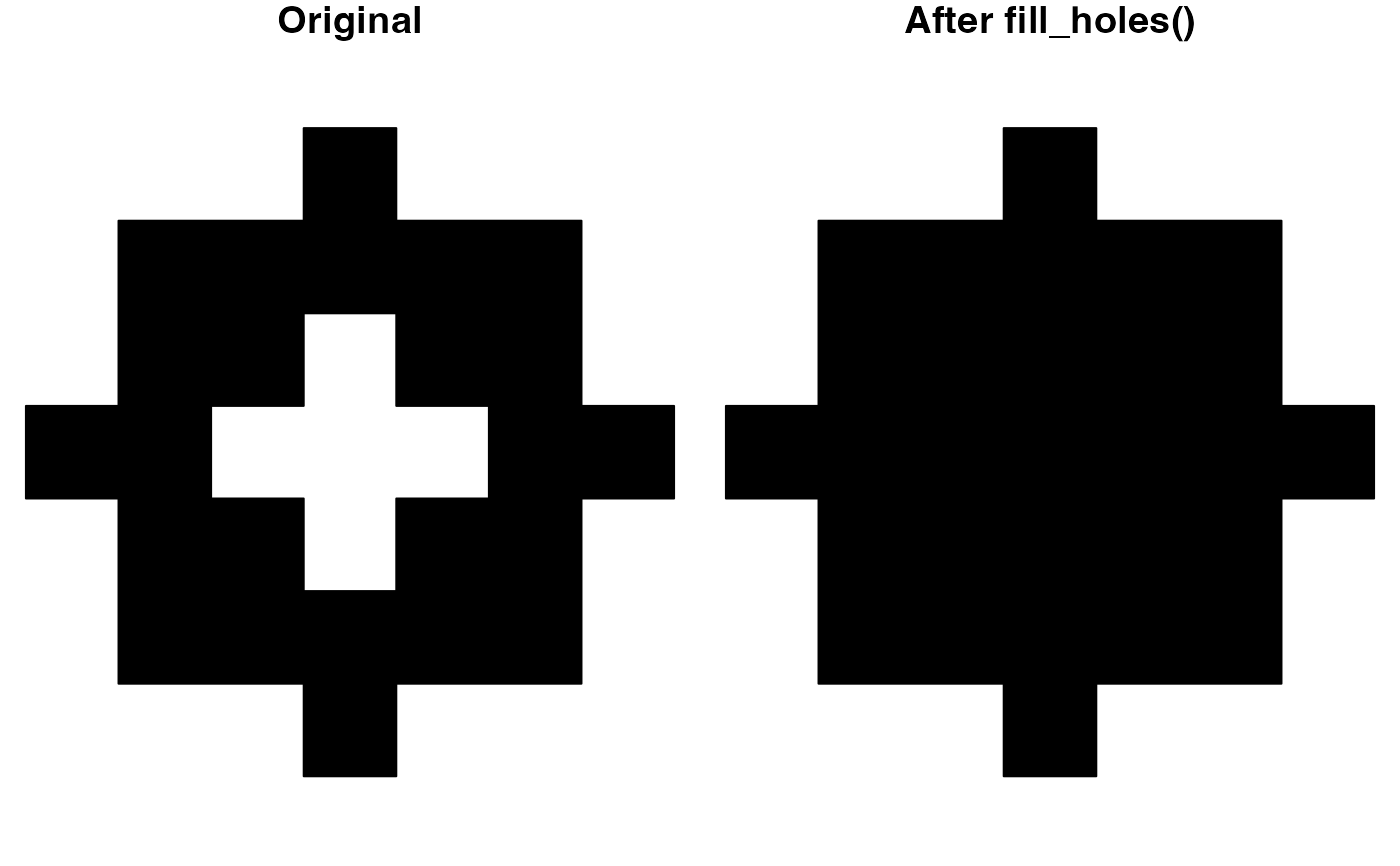Fill polygon holes that fall below a given area threshold.

fill_holes(x, threshold)

## Arguments

x spatial features; lines or polygons from either the sf, sp, or terra packages. an area threshold, below which holes will be removed. Provided either as a units object (see units::set_units()), or a numeric threshold in the units of the coordinate reference system. If x is in unprojected coordinates, a numeric threshold is assumed to be in square meters. A threshold of 0 will return the input polygons unchanged.

## Value

A spatial feature, with holes filled, in the same format as the input data.

## Examples

# fill holes smaller than 1000km2
p <- jagged_polygons\$geometry
area_thresh <- units::set_units(1000, km^2)
p_dropped <- fill_holes(p, threshold = area_thresh)
# plot
par(mar = c(0, 0, 1, 0), mfrow = c(1, 2))
plot(p, col = "black", main = "Original")
plot(p_dropped, col = "black", main = "After fill_holes()")